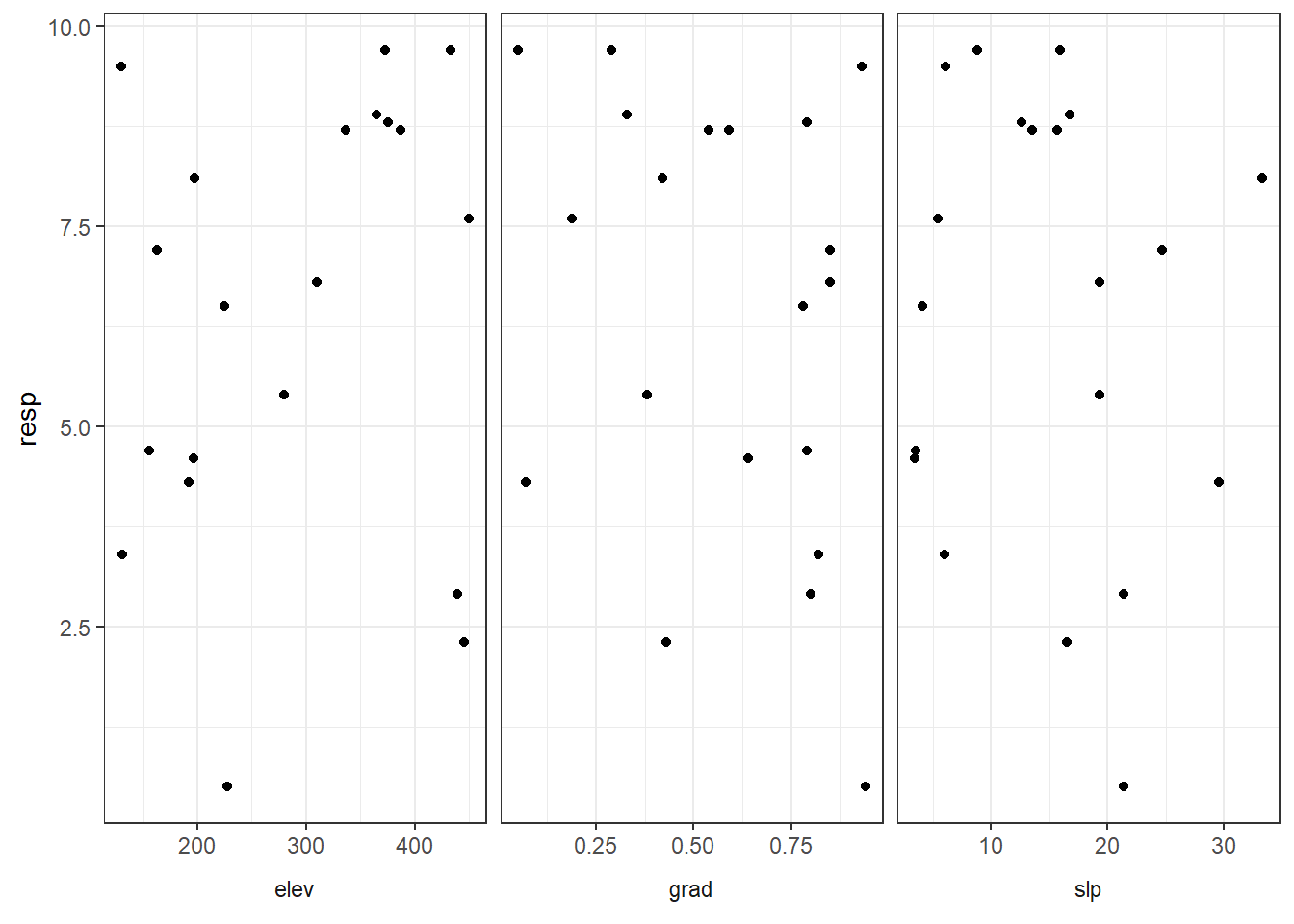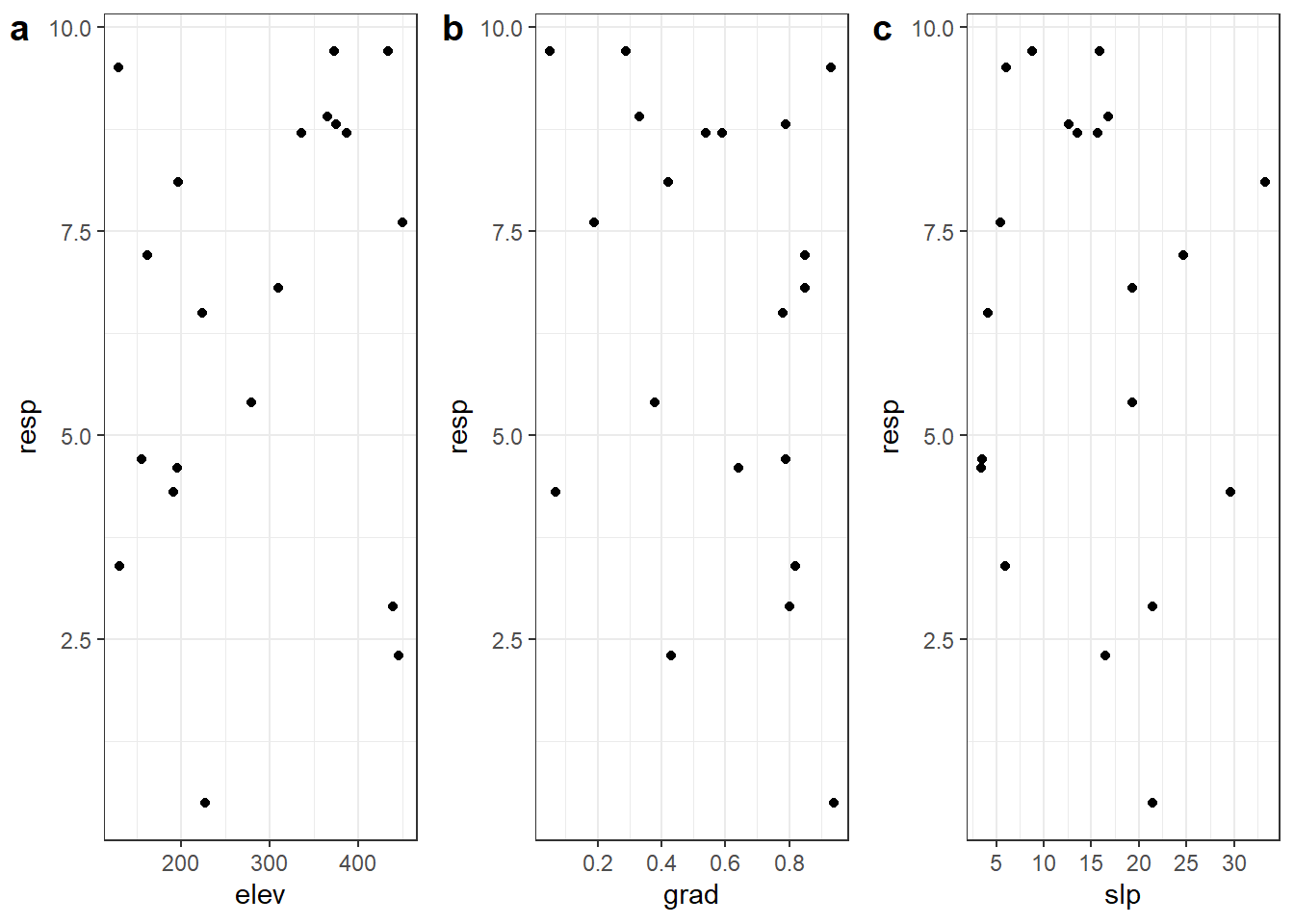# The small multiples plot: how to combine ggplot2 plots with one shared axis

Want to share your content on R-bloggers? click here if you have a blog, or here if you don't.

There are a variety of ways to combine ggplot2 plots with a single shared axis. However, things can get tricky if you want a lot of control over all plot elements.

I demonstrate three different approaches for this:
1. Using facets, which is built in to ggplot2 but doesn’t allow much control over the non-shared axes.
2. Using package cowplot, which has a lot of nice features but the plot spacing doesn’t play well with a single shared axis.
3. Using package egg, which allows nice spacing for plots with a shared axis.

I’ll be plotting with ggplot2, reshaping with tidyr, and combining plots with package egg.

I’ll also be using package cowplot version 0.9.4 to combine individual plots into one, but will use the package functions via `cowplot::` instead of loading the package. (I believe the next version of cowplot will not be so opinionated about the theme.)

``````library(ggplot2) # v. 3.1.1
library(tidyr) # v. 0.8.3
library(egg) # v. 0.4.2``````

# The set-up

Here’s the scenario: we have one response variable (`resp`) that we want to plot against three other variables and combine them into a single “small multiples” plot.

I’ll call the three variables `elev`, `grad`, and `slp`. You’ll note that I created these variables to have very different scales.

``````set.seed(16)
dat = data.frame(elev = round( runif(20, 100, 500), 1),
resp = round( runif(20, 0, 10), 1),
grad = round( runif(20, 0, 1), 2),
slp = round( runif(20, 0, 35),1) )
``````#    elev resp grad  slp
# 1 373.2  9.7 0.05  8.8
# 2 197.6  8.1 0.42 33.3
# 3 280.0  5.4 0.38 19.3
# 4 191.8  4.3 0.07 29.6
# 5 445.4  2.3 0.43 16.5
# 6 224.5  6.5 0.78  4.1``````

# Using facets for small multiples

One good option when we want to make a similar plot for different groups (in this case, different variables) is to use faceting to make different panels within the same plot.

Since the three variables are currently in separate columns we’ll need to reshape the dataset prior to plotting. I’ll use `gather()` from tidyr for this.

``````datlong = gather(dat, key = "variable", value = "value", -resp)
``````#   resp variable value
# 1  9.7     elev 373.2
# 2  8.1     elev 197.6
# 3  5.4     elev 280.0
# 4  4.3     elev 191.8
# 5  2.3     elev 445.4
# 6  6.5     elev 224.5``````

Now I can use `facet_wrap()` to make a separate scatterplot of `resp` vs each variable. The argument `scales = "free_x"` allows the x axis scales to differ for each variable but leaves a single y axis.

``````ggplot(datlong, aes(x = value, y = resp) ) +
geom_point() +
theme_bw() +
facet_wrap(~variable, scales = "free_x")``````I can use the facet strips to give the appearance of axis labels, as shown in this Stack Overflow answer.

``````ggplot(datlong, aes(x = value, y = resp) ) +
geom_point() +
theme_bw() +
facet_wrap(~variable, scales = "free_x", strip.position = "bottom") +
theme(strip.background = element_blank(),
strip.placement = "outside") +
labs(x = NULL)``````That’s a pretty nice plot to start with. However, controlling the axis breaks in the individual panels can be complicated, which is something we’d commonly want to do.

In that case, it may make more sense to create separate plots and then combine them into a small multiples plot with an add-on package.

# Using cowplot to combine plots

Package cowplot is a really nice package for combining plots, and has lots of bells and whistles along with some pretty thorough vignettes.

The first step is to make each of the three plots separately. If doing lots of these we’d want to use some sort of loop to make a list of plots as I’ve demonstrated previously. Today I’m going to make the three plots manually.

``````elevplot = ggplot(dat, aes(x = elev, y = resp) ) +
geom_point() +
theme_bw()

geom_point() +
theme_bw() +
scale_x_continuous(breaks = seq(0, 1, by = 0.2) )

slpplot = ggplot(dat, aes(x = slp, y = resp) ) +
geom_point() +
theme_bw() +
scale_x_continuous(breaks = seq(0, 35, by = 5) )``````

The function `plot_grid()` in cowplot is for combining plots. To make a single row of plots I use `nrow = 1`.

The `labels` argument puts separate labels on each panel for captioning.

``````cowplot::plot_grid(elevplot,
slpplot,
nrow = 1,
labels = "auto")``````But we want a single shared y axis, not a separate y axis on each plot. I’ll remake the combined plot, this time removing the y axis elements from all but the first plot.

``````cowplot::plot_grid(elevplot,
axis.ticks.y = element_blank(),
axis.title.y = element_blank() ),
slpplot + theme(axis.text.y = element_blank(),
axis.ticks.y = element_blank(),
axis.title.y = element_blank() ),
nrow = 1,
labels = "auto")``````This makes panels different sizes, though, which isn’t ideal. To have all the plots the same width I need to align them vertically with `align = "v"`.

``````cowplot::plot_grid(elevplot,
theme(axis.text.y = element_blank(),
axis.ticks.y = element_blank(),
axis.title.y = element_blank() ),
slpplot +
theme(axis.text.y = element_blank(),
axis.ticks.y = element_blank(),
axis.title.y = element_blank() ),
nrow = 1,
labels = "auto",
align = "v")``````But, unfortunately, this puts the axis space back between the plots to make them all the same width. It turns out that cowplot isn’t really made for plots with a single shared axis. The cowplot package author points us to package egg for this in this Stack Overflow answer.

# Using egg to combine plots

Package egg is another nice alternative for combining plots into a small multiples plot. The function in this package for combining plots is called `ggarrange()`.

Here are the three plots again.

``````elevplot = ggplot(dat, aes(x = elev, y = resp) ) +
geom_point() +
theme_bw()

geom_point() +
theme_bw() +
scale_x_continuous(breaks = seq(0, 1, by = 0.2) )

slpplot = ggplot(dat, aes(x = slp, y = resp) ) +
geom_point() +
theme_bw() +
scale_x_continuous(breaks = seq(0, 35, by = 5) )``````

The `ggarrange()` function has an `nrow` argument so I can keep the plots in a single row.

The panel spacing is automagically the same here after I remove the y axis elements, and things look pretty nice right out of the box.

``````ggarrange(elevplot,
theme(axis.text.y = element_blank(),
axis.ticks.y = element_blank(),
axis.title.y = element_blank() ),
slpplot +
theme(axis.text.y = element_blank(),
axis.ticks.y = element_blank(),
axis.title.y = element_blank() ),
nrow = 1)``````We can bring panes closer by removing some of the space around the plot margins with the `plot.margin` in `theme()`. I’ll set the spacing for right margin of the first plot, both left and right margins of the second, and the left margin of the third.

``````ggarrange(elevplot +
theme(axis.ticks.y = element_blank(),
plot.margin = margin(r = 1) ),
theme(axis.text.y = element_blank(),
axis.ticks.y = element_blank(),
axis.title.y = element_blank(),
plot.margin = margin(r = 1, l = 1) ),
slpplot +
theme(axis.text.y = element_blank(),
axis.ticks.y = element_blank(),
axis.title.y = element_blank(),
plot.margin = margin(l = 1)  ),
nrow = 1)``````# Adding plot labels with tag_facet() in egg

You’ll see there is a `labels` argument in `ggarrange()` documentation, but it didn’t work well for me out of the box with only one plot with a y axis. However, we can get tricky with `egg::tag_facet()` if we add a facet strip to each of the individual plots.

It’d make sense to build these plots outside of `ggarrange()` and then add the tags and combine them instead of nesting everything like I did here, since the code is now a little hard to follow.

``````ggarrange(tag_facet(elevplot +
theme(axis.ticks.y = element_blank(),
plot.margin = margin(r = 1) ) +
facet_wrap(~"elev"),
tag_pool = "a"),
theme(axis.text.y = element_blank(),
axis.ticks.y = element_blank(),
axis.title.y = element_blank(),
plot.margin = margin(r = 1, l = 1) ) +
tag_pool = "b" ),
tag_facet(slpplot +
theme(axis.text.y = element_blank(),
axis.ticks.y = element_blank(),
axis.title.y = element_blank(),
plot.margin = margin(l = 1)  ) +
facet_wrap(~"slp"),
tag_pool = "c"),
nrow = 1)``````We might want to add a right y axis to the right-most plot. In this case we’d want to change the axis ticks length to 0 via `theme()` elements. This can be done separately per axis in the development version of ggplot2, and will be included in version 3.2.0.

Here’s the code without all the discussion.

``````library(ggplot2) # v. 3.1.1
library(tidyr) # v. 0.8.3
library(egg) # v. 0.4.2

set.seed(16)
dat = data.frame(elev = round( runif(20, 100, 500), 1),
resp = round( runif(20, 0, 10), 1),
grad = round( runif(20, 0, 1), 2),
slp = round( runif(20, 0, 35),1) )

datlong = gather(dat, key = "variable", value = "value", -resp)

ggplot(datlong, aes(x = value, y = resp) ) +
geom_point() +
theme_bw() +
facet_wrap(~variable, scales = "free_x")

ggplot(datlong, aes(x = value, y = resp) ) +
geom_point() +
theme_bw() +
facet_wrap(~variable, scales = "free_x", strip.position = "bottom") +
theme(strip.background = element_blank(),
strip.placement = "outside") +
labs(x = NULL)

elevplot = ggplot(dat, aes(x = elev, y = resp) ) +
geom_point() +
theme_bw()

geom_point() +
theme_bw() +
scale_x_continuous(breaks = seq(0, 1, by = 0.2) )

slpplot = ggplot(dat, aes(x = slp, y = resp) ) +
geom_point() +
theme_bw() +
scale_x_continuous(breaks = seq(0, 35, by = 5) )

cowplot::plot_grid(elevplot,
slpplot,
nrow = 1,
labels = "auto")

cowplot::plot_grid(elevplot,
axis.ticks.y = element_blank(),
axis.title.y = element_blank() ),
slpplot + theme(axis.text.y = element_blank(),
axis.ticks.y = element_blank(),
axis.title.y = element_blank() ),
nrow = 1,
labels = "auto")

cowplot::plot_grid(elevplot,
theme(axis.text.y = element_blank(),
axis.ticks.y = element_blank(),
axis.title.y = element_blank() ),
slpplot +
theme(axis.text.y = element_blank(),
axis.ticks.y = element_blank(),
axis.title.y = element_blank() ),
nrow = 1,
labels = "auto",
align = "v")

elevplot = ggplot(dat, aes(x = elev, y = resp) ) +
geom_point() +
theme_bw()

geom_point() +
theme_bw() +
scale_x_continuous(breaks = seq(0, 1, by = 0.2) )

slpplot = ggplot(dat, aes(x = slp, y = resp) ) +
geom_point() +
theme_bw() +
scale_x_continuous(breaks = seq(0, 35, by = 5) )

ggarrange(elevplot,
theme(axis.text.y = element_blank(),
axis.ticks.y = element_blank(),
axis.title.y = element_blank() ),
slpplot +
theme(axis.text.y = element_blank(),
axis.ticks.y = element_blank(),
axis.title.y = element_blank() ),
nrow = 1)

ggarrange(elevplot +
theme(axis.ticks.y = element_blank(),
plot.margin = margin(r = 1) ),
theme(axis.text.y = element_blank(),
axis.ticks.y = element_blank(),
axis.title.y = element_blank(),
plot.margin = margin(r = 1, l = 1) ),
slpplot +
theme(axis.text.y = element_blank(),
axis.ticks.y = element_blank(),
axis.title.y = element_blank(),
plot.margin = margin(l = 1)  ),
nrow = 1)

ggarrange(tag_facet(elevplot +
theme(axis.ticks.y = element_blank(),
plot.margin = margin(r = 1) ) +
facet_wrap(~"elev"),
tag_pool = "a"),
theme(axis.text.y = element_blank(),
axis.ticks.y = element_blank(),
axis.title.y = element_blank(),
plot.margin = margin(r = 1, l = 1) ) +
tag_pool = "b" ),
tag_facet(slpplot +
theme(axis.text.y = element_blank(),
axis.ticks.y = element_blank(),
axis.title.y = element_blank(),
plot.margin = margin(l = 1)  ) +
facet_wrap(~"slp"),
tag_pool = "c"),
nrow = 1)``````

R-bloggers.com offers daily e-mail updates about R news and tutorials about learning R and many other topics. Click here if you're looking to post or find an R/data-science job.
Want to share your content on R-bloggers? click here if you have a blog, or here if you don't.